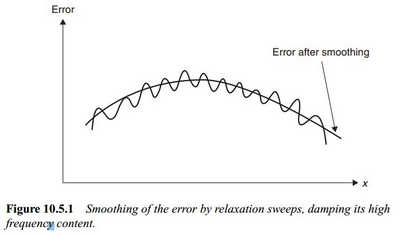# 有关preditioner和smoother

• OpenFOAM里面preconditioner和smother是用来干嘛的？

• Hi，

## 我们用方程举例：加入你要求解矩阵： \begin{equation} A \phi = b \end{equation} 直接解法是不能用了大家都知道。一般采用迭代解法。在迭代解法中，如果对矩阵操作一下： \begin{equation} A = M-N \end{equation} 替换到公式(1)中，就是 \begin{equation} \phi^n = M^{-1} N \phi^{n-1} + M^{-1}b \end{equation} 这种操作就是预条件，依据不同的M，有不同的预条件方法。OpenFOAM里面采用不完全LU分解。

smoother更倾向是多重网格矩阵求解器的内容，如图：多重网格这块暂时还没涉及，以后有涉及随时补充。

推荐看这本书：Numerical Computation of Internal and External Flows - Volume I - Fundamentals of Numerical Discretization